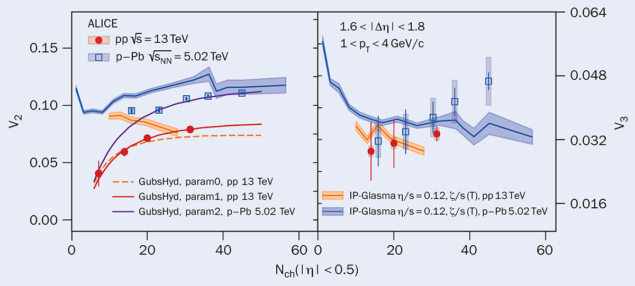# Collectivity in small systems produced at the LHC

3 November 2023

A report from the ALICE experiment.Fig. 1. The measured and calculated evolution of elliptic (left) and triangular (right) flow in pp and pPb collisions as a function of charged-particle multiplicity at midrapidity. The measurements are compared to the state-of-the-art hydrodynamic calculations. Source: arXiv:2308.16591

High-energy heavy-ion collisions at the LHC exhibit strong collective flow effects in the azimuthal angle distribution of final-state particles. Since these effects are governed by the initial collision geometry of the two colliding nuclei and the hydrodynamic evolution of the collision, the study of anisotropic flow is a powerful way to characterise the production of the quark–gluon plasma (QGP) – an extreme state of matter expected to have existed in the early universe.

To their surprise, researchers on the ALICE experiment have now revealed similar flow signatures in small systems encompassing proton–proton (pp) and proton–lead (pPb) collisions, where QGP formation was previously assumed not to occur. The origin of the flow signals in small systems (and in particular whether the mechanisms behind these correlations in small systems share commonalities with heavy-ion collisions) are not yet fully understood. To better interpret these results, and thus to understand the limit of the system size that exhibits fluid-like behaviour, it is important to carefully single out possible scenarios that can mimic the effect of collective flow.

Anisotropic-flow measurements become more difficult in small systems because non-flow effects, such as the presence of jets, become more dominant. Thus, it is important to examine methods where non-flow effects are properly subtracted first. One of the methods, the so-called low-multiplicity template fit, has been widely used in several experiments to determine and subtract the non-flow elements.

The origin of the flow signals in small systems is not yet fully understood

The ALICE collaboration studied long-range angular correlations for pairs of charged particles produced in pp and pPb collisions at centre-of-mass energies of 13 TeV and 5.02 TeV, respectively. Flow coefficients were extracted from these correlations using the template-fit method in samples of events with different charged-particle multiplicities. This method considers that the yield of jet fragments increases as a function of particle multiplicity and allows physicists to examine assumptions made in the low-multiplicity template fit for the first time – demonstrating their validity, including a possible jet-shape modification.

Figure 1 shows the measurement of two components of anisotropic flow – elliptic (v2) and triangular (v3) – as a function of charged-particle multiplicity at midrapidity (Nch). The data show decreasing trends towards lower multiplicities. In pp collisions, the results suggest that the v2 signal disappears below Nch = 10. The results are then compared with hydrodynamic models. To accurately describe the data, especially for events with low multiplicities, a better understanding of initial conditions is needed.

These results can help to constrain the modelling of initial-state simulations, as the significance of initial-state effects increases for collisions resulting in low multiplicities. The measurements with larger statistics from Run 3 data will push down this multiplicity limit and reduce the associated uncertainties.